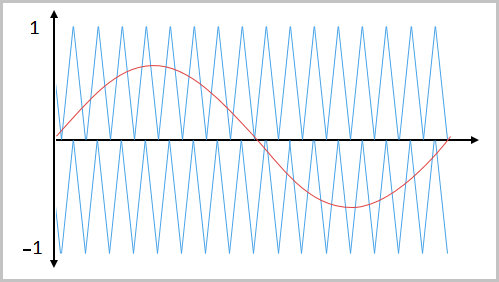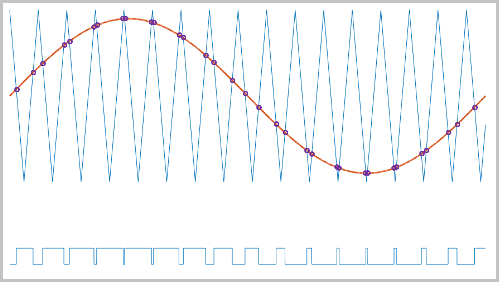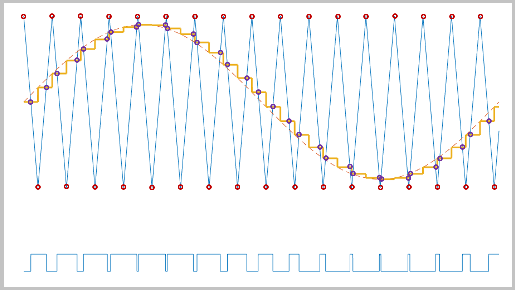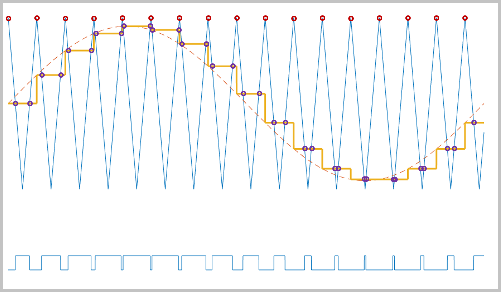# PWM Timing and Waveform Generator (Three-phase, Three-level)

Generate three-phase, three-level gating time information

Since R2020b

•Libraries:
Simscape / Electrical / Control / Pulse Width Modulation

## Description

The PWM Timing and Waveform Generator (Three-phase, Three-level) block controls switching behavior for a three-phase, three-level power converter. The block calculates on-gating and off-gating times and modulation waves based on these block inputs:

• Three sinusoidal reference voltages

• A DC-link neutral point balance control signal

This block can be used with average converters to speed up the simulation.

### Sampling Mode

This block allows you to choose natural, symmetric, or asymmetric sampling of the modulation wave.

The PWM Timing and Waveform Generator (Three-phase, Three-level) block does not perform carrier-based pulse width modulation (PWM). Instead, the block uses input signals to calculate the gating times.

Carrier-based PWM is, however, useful for showing how the sampling mode that you select relates to the switch-on and switch-off behavior of the pulses that the block generates. A generator that uses a three-level, carrier-based PWM method:

1. Samples a reference wave

2. Compares the sample to two parallel triangle carrier waves, separated by one level3. Generates a switch-on pulse if a sample is higher than the carrier signal or a switch-off pulse if a sample is lower than the carrier wave

To determine switch-on and switch-off pulse behavior, a three-level carrier-based PWM generator uses these methods to sample each of the triangle waves:

• Natural — The sampling and comparison occur at the intersection points of the modulation wave and the carrier wave.• Asymmetric — Sampling occurs at the upper and lower boundaries of the carrier wave. The comparison occurs at the intersection that follows the sampling.• Symmetric — Sampling occurs only at the upper boundary of the carrier wave. The comparison occurs at the intersection that follows the sampling.## Ports

### Input

expand all

Sinusoidal voltages that you want the attached converter to output, specified as a vector of three elements, with one element for each phase.

Positive real number for the DC-link voltage of the converter, specified as a scalar.

Output from a feedback-control loop that balances the DC supply, specified as a real number between `–1` and `+1`.

### Output

expand all

Switch-on gating time, returned as a vector of three elements, with one element for each phase.

Switch-off gating time, returned as a vector of three elements, with one element for each phase.

Number of the small hexagon that composes the space–vector diagrams of conventional two-level inverters, returned as a scalar between 1 and 6. For more information, see the Simplified Space-Vector PWM Method section in the PWM Gate Signal Generator (Three-phase, Three-level) block.

Modulation waves, returned as a vector of three elements, with one element for each phase.

## Parameters

expand all

Specify the waveform technique.

Wave-sampling method. The sampling mode determines whether the block samples the modulation waveform when the waves intersect or when the carrier wave is at one or both of its boundary conditions.

Rate at which the switches in the power converter switch.

 Chung, D. W., J. S. Kim, and S. K. Sul. “Unified Voltage Modulation Technique for Real Time Three-Phase Power Conversion.” IEEE Transactions on Industry Applications, Vol. 34, No. 2, 1998, pp. 374–380.

 Seo, J. H., C. H. Choi, and D. S. Hyun. “A new simplified space-vector PWM method for three-level inverters.” IEEE Transactions on Power Electronics, Vol. 16, No. 4, 2001, pp. 545-550.